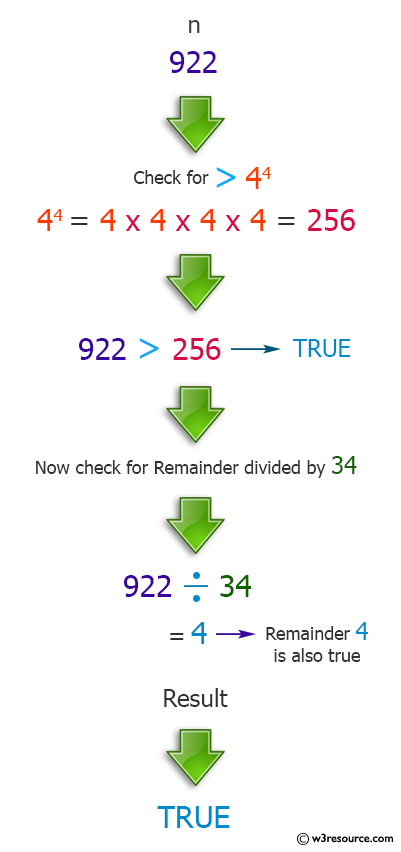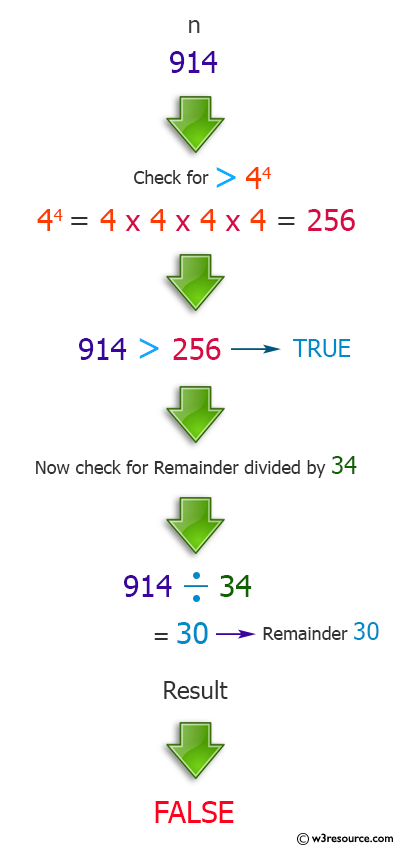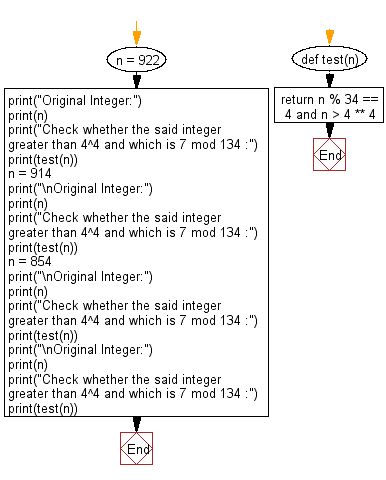﻿ Python: Whether an integer greater than 4^4 which is 4 mod 34 - w3resource# Python: Whether an integer greater than 4^4 which is 4 mod 34

## Python Programming Puzzles: Exercise-3 with Solution

Write a Python program that accept an integer test whether an integer greater than 4^4 and which is 4 mod 34.

```Input:
922
Output:
True

Input:
914
Output:
False

Input:
854
Output:
True

Input:
854
Output:
True
```

Pictorial Presentation:Sample Solution:

Python Code:

``````#License: https://bit.ly/3oLErEI

def test(n):
return n % 34 == 4 and n > 4 ** 4

n = 922
print("Original Integer:")
print(n)
print("Check whether the said integer greater than 4^4 and which is 7 mod 134 :")
print(test(n))
n = 914
print("\nOriginal Integer:")
print(n)
print("Check whether the said integer greater than 4^4 and which is 7 mod 134 :")
print(test(n))
n = 854
print("\nOriginal Integer:")
print(n)
print("Check whether the said integer greater than 4^4 and which is 7 mod 134 :")
print(test(n))
print("\nOriginal Integer:")
print(n)
print("Check whether the said integer greater than 4^4 and which is 7 mod 134 :")
print(test(n))
``````

Sample Output:

```Original Integer:
922
Check whether the said integer greater than 4^4 and which is 7 mod 134 :
True

Original Integer:
914
Check whether the said integer greater than 4^4 and which is 7 mod 134 :
False

Original Integer:
854
Check whether the said integer greater than 4^4 and which is 7 mod 134 :
True

Original Integer:
854
Check whether the said integer greater than 4^4 and which is 7 mod 134 :
True
```

Flowchart:## Visualize Python code execution:

The following tool visualize what the computer is doing step-by-step as it executes the said program:

Python Code Editor :

Have another way to solve this solution? Contribute your code (and comments) through Disqus.

What is the difficulty level of this exercise?

Test your Programming skills with w3resource's quiz.

﻿

## Python: Tips of the Day

Clamps num within the inclusive range specified by the boundary values x and y:

Example:

```def tips_clamp_num(num,x,y):
return max(min(num, max(x, y)), min(x, y))
print(tips_clamp_num(2, 4, 6))
print(tips_clamp_num(1, -1, -6))
```

Output:

```4
-1
```